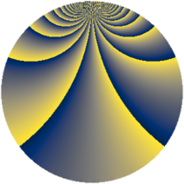# Properties

 Label 637.2.blLevel $637$ Weight $2$ Character orbit 637.bl Rep. character $\chi_{637}(53,\cdot)$ Character field $\Q(\zeta_{21})$ Dimension $672$ Newform subspaces $2$ Sturm bound $130$ Trace bound $1$

# Related objects

## Defining parameters

 Level: $$N$$ $$=$$ $$637 = 7^{2} \cdot 13$$ Weight: $$k$$ $$=$$ $$2$$ Character orbit: $$[\chi]$$ $$=$$ 637.bl (of order $$21$$ and degree $$12$$) Character conductor: $$\operatorname{cond}(\chi)$$ $$=$$ $$49$$ Character field: $$\Q(\zeta_{21})$$ Newform subspaces: $$2$$ Sturm bound: $$130$$ Trace bound: $$1$$ Distinguishing $$T_p$$: $$2$$

## Dimensions

The following table gives the dimensions of various subspaces of $$M_{2}(637, [\chi])$$.

Total New Old
Modular forms 816 672 144
Cusp forms 768 672 96
Eisenstein series 48 0 48

## Trace form

 $$672 q + 56 q^{4} + 2 q^{5} - 28 q^{6} + 6 q^{7} + 12 q^{8} + 24 q^{9} + O(q^{10})$$ $$672 q + 56 q^{4} + 2 q^{5} - 28 q^{6} + 6 q^{7} + 12 q^{8} + 24 q^{9} - 10 q^{10} - 16 q^{11} - 80 q^{12} - 4 q^{13} - 60 q^{14} - 8 q^{15} + 56 q^{16} - 18 q^{17} - 10 q^{18} - 48 q^{19} - 88 q^{20} - 2 q^{21} - 16 q^{22} - 56 q^{23} + 20 q^{24} + 42 q^{25} + 6 q^{26} - 42 q^{27} - 30 q^{28} - 48 q^{29} - 26 q^{30} - 80 q^{31} - 106 q^{32} + 8 q^{33} - 80 q^{34} + 4 q^{35} - 152 q^{36} - 8 q^{37} + 2 q^{38} + 64 q^{40} + 28 q^{41} - 32 q^{42} + 36 q^{43} - 30 q^{44} - 4 q^{45} + 156 q^{46} + 58 q^{47} + 76 q^{48} + 76 q^{49} + 60 q^{50} + 20 q^{51} + 6 q^{52} + 46 q^{53} + 186 q^{54} + 28 q^{55} - 70 q^{56} + 22 q^{57} - 54 q^{58} + 66 q^{59} - 92 q^{60} - 12 q^{61} + 104 q^{62} - 40 q^{63} - 52 q^{64} - 260 q^{66} - 16 q^{67} - 18 q^{68} - 56 q^{69} - 82 q^{70} - 22 q^{71} - 282 q^{72} + 22 q^{73} - 58 q^{74} - 230 q^{75} - 258 q^{76} + 52 q^{77} - 50 q^{78} - 26 q^{79} - 84 q^{80} - 188 q^{81} + 44 q^{82} + 58 q^{83} + 238 q^{84} + 68 q^{85} + 192 q^{86} - 56 q^{87} + 216 q^{88} + 66 q^{89} + 46 q^{90} - 8 q^{91} + 10 q^{92} + 306 q^{93} - 122 q^{94} - 46 q^{95} + 454 q^{96} + 180 q^{97} - 76 q^{98} - 104 q^{99} + O(q^{100})$$

## Decomposition of $$S_{2}^{\mathrm{new}}(637, [\chi])$$ into newform subspaces

Label Dim. $$A$$ Field CM Traces $q$-expansion
$$a_2$$ $$a_3$$ $$a_5$$ $$a_7$$
637.2.bl.a $$324$$ $$5.086$$ None $$-3$$ $$0$$ $$1$$ $$-21$$
637.2.bl.b $$348$$ $$5.086$$ None $$3$$ $$0$$ $$1$$ $$27$$

## Decomposition of $$S_{2}^{\mathrm{old}}(637, [\chi])$$ into lower level spaces

$$S_{2}^{\mathrm{old}}(637, [\chi]) \cong$$ $$S_{2}^{\mathrm{new}}(49, [\chi])$$$$^{\oplus 2}$$